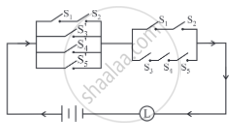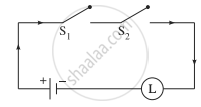# Simplify the following so that the new circuit has a minimum number of switches. Also, draw the simplified circuit. - Mathematics and Statistics

Sum

Simplify the following so that the new circuit has a minimum number of switches. Also, draw the simplified circuit.#### Solution

Let p: the switch S1 is closed
q: the switch S2 is closed
r: the switch S3 is closed
s: the switch S4 is closed
t: the switch S5 is closed
∼p: the switch S1′ is closed or the switch S1 is open
∼q: the switch S2′ is closed or the switch S2 is open
∼r: the switch S3′ is closed or the switch S3 is open
∼s: the switch S4′ is closed or the switch S4 is open
∼t: the switch S5′ is closed or the switch S5 is open.
Then the given circuit in symbolic form is:
[(p ∧ q) ∨ ∼r ∨ ∼s ∨ ∼t] ∧ [(p ∧ q) ∨ (r ∧ s ∧t )]
Using the laws of logic, we have,
[(p ∧ q) ∨ ∼r ∨ ∼s ∨ ∼t] ∧ [(p ∧ q) ∨ (r ∧ s ∧t )]
≡ [(p ∧ q) ∨ ∼ (r ∧ s ∧ t)] ∧ [(p ∧ q) ∨ (r ∧ s ∧ t)] .........(By De Morgan’s Law)
≡ (p ∧ q) ∨ [∼ (r ∧ s ∧ t) ∧ (r ∧ s ∧ t)] ......(By Distributive Law)
≡ (p ∧ q) ∨ F ...........(By Complement Law)
≡ p ∧ q .......(By Identity Law)
Hence, the alternative simplified circuit is:Concept: Application of Logic to Switching Circuits
Is there an error in this question or solution?

#### APPEARS IN

Balbharati Mathematics and Statistics 1 (Arts and Science) 12th Standard HSC Maharashtra State Board
Chapter 1 Mathematical Logic
Miscellaneous Exercise 1 | Q 13.2 | Page 34